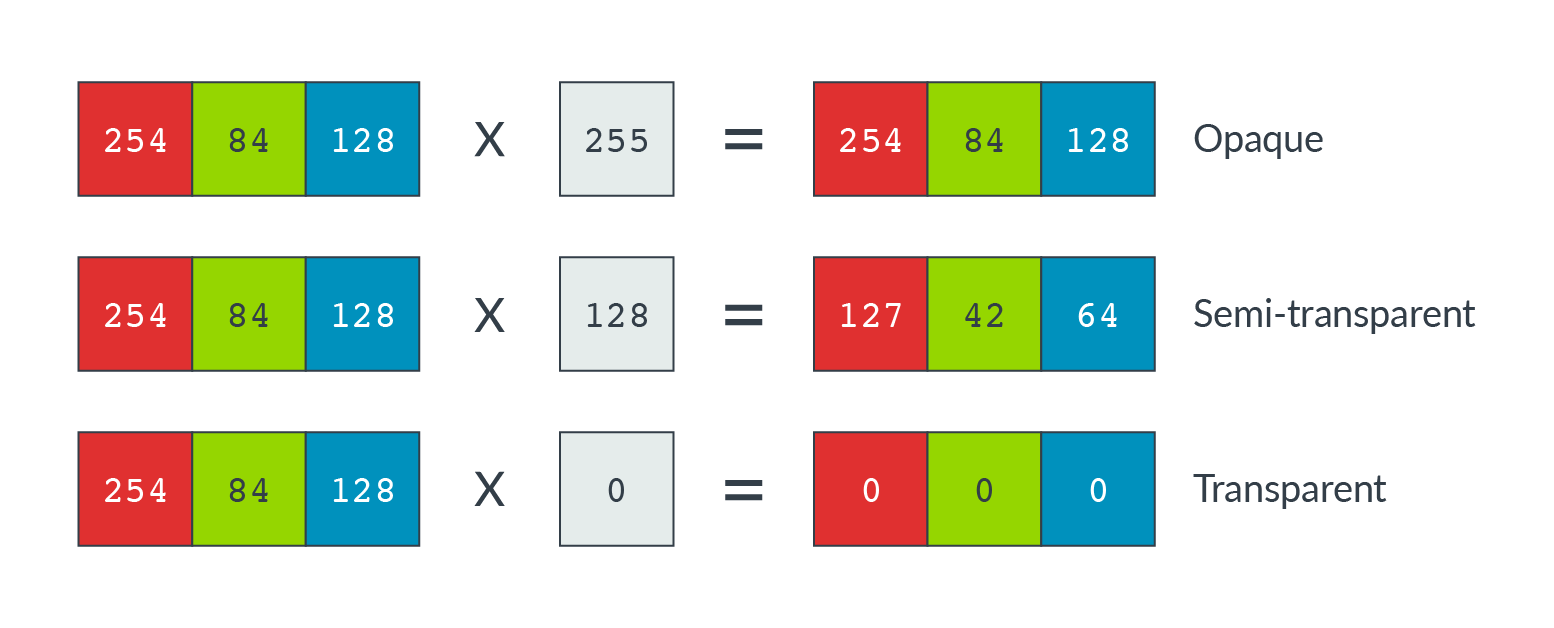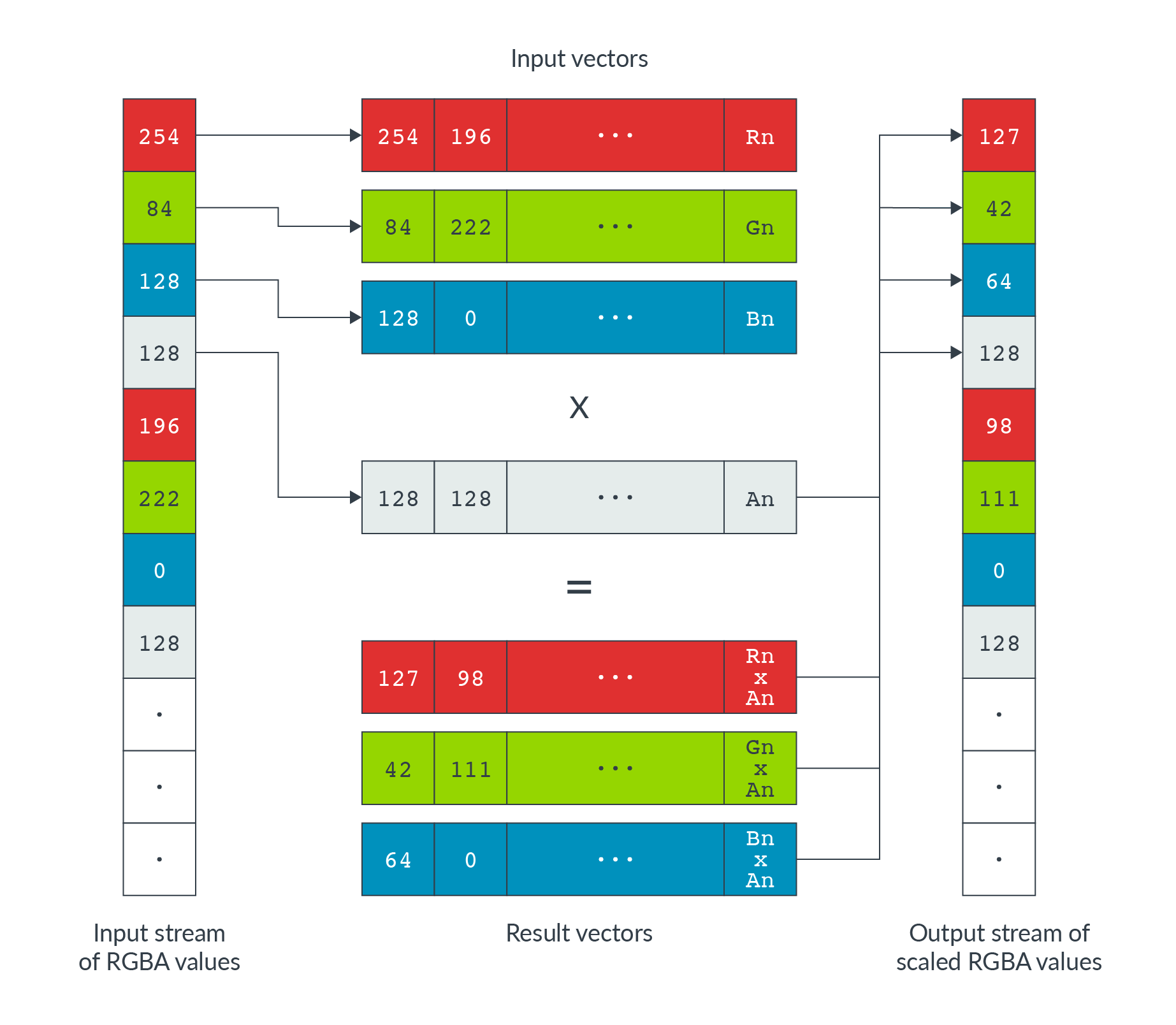## Pre-multiplied alpha channel data

The color of each pixel in a PNG image is defined by an RGB triple. An additional value, called the alpha channel, specifies the opacity of the pixel. Each of the R, G, B, and A values are integers between 0 and 255. An alpha value of 0 means the pixel is transparent and does not appear in the final image. A value of 255 means the pixel is totally opaque and obscures any other image data in the same location.

When rendering a PNG image, the browser needs to calculate pre-multiplied alpha data. That is, the RGB data for each pixel must be multiplied by the corresponding alpha channel value. This calculation produces scaled RGB data that accounts for the opacity of the pixel.

The following diagram shows the same RGB pixel scaled by three different alpha values:Each scaled color value is calculated as you can see in the following code:

`Scaled_RGB_value = straight_rgb_value x (alpha_value / 255)`

### Unoptimized implementation

In Chromium, the code that performs this calculation is the `ImageFrame::setRGBAPremultiply()` function. Before Neon optimization, this function had the following implementation:

```static inline void setRGBAPremultiply(PixelData* dest,
unsigned r,
unsigned g,
unsigned b,
unsigned a) {
enum FractionControl { RoundFractionControl = 257 * 128 };

if (a < 255) {
unsigned alpha = a * 257;
r = (r * alpha + RoundFractionControl) >> 16;
g = (g * alpha + RoundFractionControl) >> 16;
b = (b * alpha + RoundFractionControl) >> 16;
}

*dest = SkPackARGB32NoCheck(a, r, g, b);
}```

This unoptimized function operates on a single RGBA value at a time, multiplying each of the R, G, and B values by the alpha channel.

### Neon-optimized implementation

The serial data processing performed in the unoptimized implementation provides an opportunity for Neon optimization. Rather than operating on a single data value at a time, we can:

• Load the RGBA data into separate R, G, B and A input vectors. Use a de-interleaved load. In this case, that means loading every fourth data value into the same register.
• Multiply each data lane with its corresponding alpha value simultaneously.
• Store the scaled data with an interleaved store. This means storing values from each of the four registers into adjacent memory locations, to produce an output stream of scaled RGBA data.The Neon optimized code is as follows:

```static inline void SetRGBAPremultiplyRowNeon(png_bytep src_ptr,
const int pixel_count,
ImageFrame::PixelData* dst_pixel,
// Input registers.
uint8x8x4_t rgba;

// Scale the color channel by alpha - the opacity coefficient.
auto premultiply = [](uint8x8_t c, uint8x8_t a) {
// First multiply the color by alpha, expanding to 16-bit (max 255*255).
uint16x8_t ca = vmull_u8(c, a);
// Now we need to round back down to 8-bit, returning (x+127)/255.
// (x+127)/255 == (x + ((x+128)>>8) + 128)>>8.  This form is well suited
// to NEON: vrshrq_n_u16(...,8) gives the inner (x+128)>>8, and
// vraddhn_u16() both the outer add-shift and our conversion back to 8-bit.
};

.
.
.

// Main loop

rgba = vld4_u8(src_ptr);

// Premultiply with alpha channel
rgba.val = premultiply(rgba.val, rgba.val);
rgba.val = premultiply(rgba.val, rgba.val);
rgba.val = premultiply(rgba.val, rgba.val);

// Write back (interleaved) results to memory.
vst4_u8(reinterpret_cast<uint8_t*>(dst_pixel), rgba);

}```

Intrinsic Description
`vmull_u8` Vector multiply.
`vraddhn_u16` Vector rounding addition.
`vrshrq_n_u16` Vector rounding shift right.
`vld4_u8` Load multiple 4-element structures to four vector registers.
`vst4_u8` Store multiple 4-element structures from four vector registers.

### Results

This optimization gave results in the region of 9% improvement.

The following resources provide additional information about the `ImageFrame::setRGBAPremultiply()` optimization: